Figure   212.   Removing   reverse-range   planetary   carrier assembly
Custom Search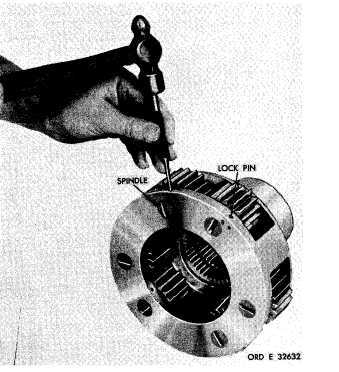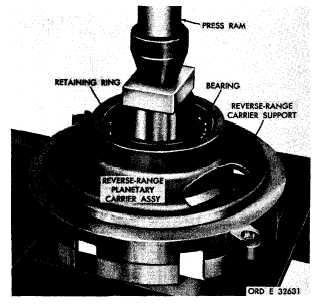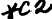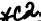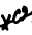*C2 *C2 *C2 PAR  177-181 R E V E R S E     P L A N E T A R Y     A N D     S U P P O R T     R E B U I LD C H A P    5,   SEC  X X I II S e c t i o n    X X I I I .    R E V E R S E - R A N G E    P L A N E T A R Y    A N D    S U P P O R T    A S S E M B L Y    —    R E B U I LD 1 7 7 .    D E S C R I P T I ON Refer   to   par.   14   for   description   of   the   re- verse-range    planetary    and    support    assembly. 1 7 8 .    D I S A S S E M B L Y    ( f i g .    3 7 7 ,    f o l d - o u t    6) .   Using   a   press,   remove   the   reverse- r a n g e    p l a n e t a r y    c a r r i e r    a s s e m b l y    f r o m    i t s support   (fig.   212). .   R e m o v e    t h e    r e t a i n i n g    r i n g    r e t a i n i ng the   bearing   in   the   carrier   support   (fig.   212). .   U s i n g    a    p r e s s ,    r e m o v e    t h e    b e a r i n g from   the   carrier   support   (fig.   212). .   U s i n g    a    h a m m e r    a n d    p u n c h ,    r e m o ve six   lock   pins   retaining   the   planetary   carrier spindles   (fig.   213). .   P o s i t i o n    t h e    r e v e r s e - r a n g e    p l a n e t a ry carrier   assembly   in   a   press.   Using   a   suitable p r e s s i n g    t o o l ,    r e m o v e    s i x    c a r r i e r    s p i n d l e s ( f i g .    2 1 4 ) .    R e m o v e    t h e    p i n i o n s    1 4    ( f i g .    3 7 7, fold-out   6),   thrust   washers   8   and   15,   spacers 9,   11   and   13,   and   roller   bearings   10   and   12. Figure   212.   Removing   reverse-range   planetary   carrier assembly 1 7 9 .     C L E A N I NG Refer   to   par.   71   for   cleaning   recommen- dations. 1 8 0 .    I N S P E C T I O N    A N D    R E P A IR R e f e r    t o    p a r .    7 2    f o r    g e n e r a l    i n s p e c t i on and    repair    recommendations.    Repair    and    re- b u i l d    p o i n t s    o f    m e a s u r e m e n t    f o r    f i t s ,    c l e a r- ances   and   wear   limits   are   indicated   by   small, lower   case   letters   in   fig.   377,   fold-out   6.   Re- fer   to   par.   240   for   wear   limits   information. 1 8 1 .    A S S E M B L Y    ( f i g .    3 7 7 ,    f o l d - o u t    6) .   Chill   spindles   7   in   dry   ice   for approximately   one   hour,   prior   to   in- stallation. .   P l a c e    r e v e r s e - r a n g e    p l a n e t a r y    c a r- rier   17,   hub   side   down,   in   a   press. Figure  213.  Removing  (or  installing)  spindle  lock  pins 1 4 7Integrated Publishing, Inc. - A (SDVOSB) Service Disabled Veteran Owned Small Business